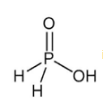Courses

# Test: p-Block Elements- 1

## 25 Questions MCQ Test Chemistry Class 12 | Test: p-Block Elements- 1

Description
This mock test of Test: p-Block Elements- 1 for JEE helps you for every JEE entrance exam. This contains 25 Multiple Choice Questions for JEE Test: p-Block Elements- 1 (mcq) to study with solutions a complete question bank. The solved questions answers in this Test: p-Block Elements- 1 quiz give you a good mix of easy questions and tough questions. JEE students definitely take this Test: p-Block Elements- 1 exercise for a better result in the exam. You can find other Test: p-Block Elements- 1 extra questions, long questions & short questions for JEE on EduRev as well by searching above.
QUESTION: 1

### Which among the following compound, nitrogen shows the oxidation state of +5?

Solution:

N has +5 in HNO3. Oxidation state of O is -2 and H is +1.​

QUESTION: 2

### Which among the following forms basic oxide?

Solution:

As we move down the group metallic character increases so Bi is a metal thus its oxide is basic.

QUESTION: 3

### In laboratory ammonia is prepared by heating

Solution:

2(NH4Cl) + Ca(OH)2 → (CaCl)2 + 2(NH3) + 2(H2O)
Hence C is correct.

QUESTION: 4

Ammonia has a higher boiling point and is less volatile because of

Solution:

NHundergo intermolecular hydrogen bonding and therefore it has higher boiling point compared to other hydrides of group 15.

QUESTION: 5

Which compound is used as the cooling liquid in refrigerators?

Solution:

NH3  is used as cooling liquid in refrigerators.

QUESTION: 6

At what temperature white phosphorous changes to red phosphorous?

Solution:

White phosphorus when heated to 300° C polymerization occurs and it changes to Red Phosphorus.

QUESTION: 7is the structure of

Solution:

H3PO is Hypophosphorus acid

QUESTION: 8

Elements of which group are known as ore forming elements?

Solution:

Maximum ores are of group 16 elemnts (O, S etc).

QUESTION: 9

Most metal oxides are

Solution:

Metal oxides are basic and ionic in nature.

QUESTION: 10

The stability of hydrides of group 16 elements decreases in the order

Solution:

As we move down the group bond length increases therefore stability of hydrides decreases. Order of bond length O-H < S-H < Se-H < Te-H.

QUESTION: 11

Formula of oleum is

Solution:

Oleum is H2S2O7

QUESTION: 12

Which among the following does not exhibit positive oxidation state?

Solution:

Fluorine is most electronegative element therefore can only gain electrons. Thus it only shows negative oxidation state.

QUESTION: 13

Which of the following is the strongest Lewis base?

Solution:

Explanation : Lewis bases need to be able to donate electrons. Fluorine is the most electronegative element in the halogens followed by chlorine, bromine and iodine.

Due to fluorine being strongly electronegative, it draws the electron density towards itself which makes it difficult for nitrogen atom to donate its lone pair of electrons. So, NF is the least basic. This trend follows the strength of electronegativity of the halides.

Since iodine is least electronegative, it is the most basic trihalide of nitrogen.

So, we have the trend, in decreasing order of basic strength:

NF3 < NCl3 < NBr3 < NI3

QUESTION: 14

Hydrogen sulphide reacts with lead acetate forming a black compound which reacts with hydrogen peroxide to form another compound. The colour of the compound is

Solution:

PbS + H2O2 → PbSo4(white) + H2O

QUESTION: 15

Which of the following is thermally the most stable?

Solution:

Stability of hydrides decreases down the group so most stable is H2O

QUESTION: 16

Which of the following is prepared by cyanamide process?

Solution:

CaNCN + 3H2O → CaCO+ 2NH3

QUESTION: 17

Ozone can be detected by using

Solution:

Ozone is detected by using Hg.

QUESTION: 18

Which of the following oxides reacts with both HCl and NaOH?

Solution:

ZnO is an amphroteric oxide.

QUESTION: 19

Name the gas whose formula was established by Sorret?

Solution:

Sorret established formula of ozone.

QUESTION: 20

Which colour is given by CuSO4 with ammonia?

Solution:

Cu2+  + 4NH3 → [Cu (NH3)4]2+ (blue)

QUESTION: 21

Xenondifluoride is

Solution:

CN = 0.5(V+M-C+A) For XeF2 CN = 5 so shape will be linear and structure will be trigonal pyramidal.

QUESTION: 22

A metal X on heating in nitrogen gas gives Y. Y on treatment with water gives a colourless gas which when passed through copper sulphate solution gives a blue colour. Y is

Solution:

Mg3Nreacts with water to evolve NH3. Which reacts with copper ion to form blue color complex.

QUESTION: 23

The reagent used to distinguish between H2O2 and Ois:

Solution:

H2Ocan decolorize the color of KMnO4

QUESTION: 24

When chlorine is passed through concentrated hot solution of KOH, the compound formed is

Solution:

Clon treatment with conc. Base form ClO3-

QUESTION: 25

Ammonia can be dried using

Solution:

CaO is used to dry NH3